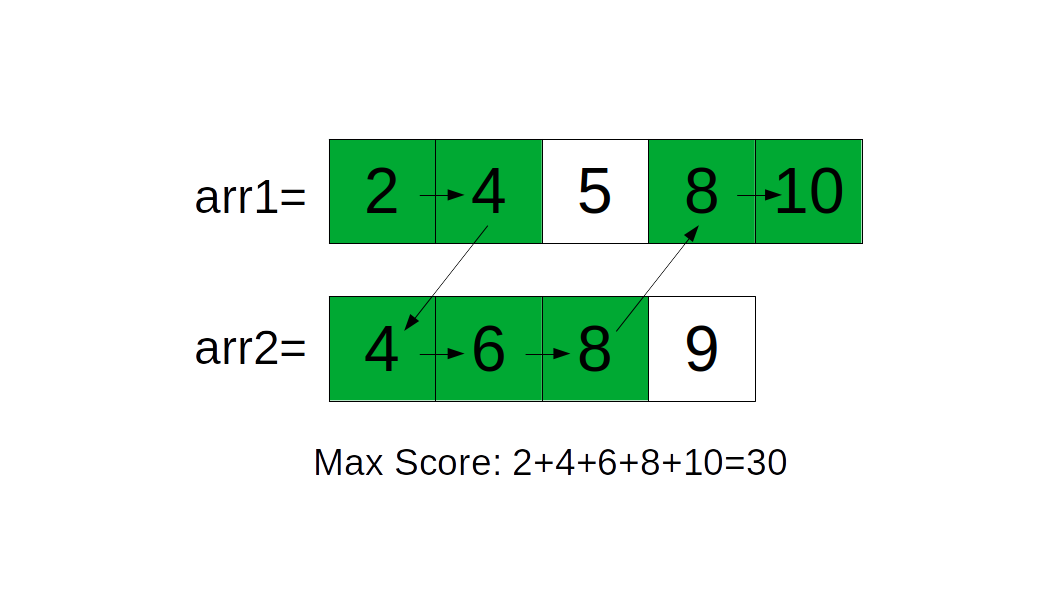# Teleporter Arrays

You are given two sorted arrays of distinct integers, `arr1`, and `arr2`. Your goal is to start from the beginning of one array, and arrive to the end of one array (it could be the same array or not).

For each step, you can either move forward a step on an array, or move to a square in the other array where the number is the same as the number in your current square ("teleport"). Your total score is defined as the sum of all unique numbers that you have been on.

Find the maximum score that you can get given the above rules. Since the result might be very large and cause overflow, return the maximum score modded by `10^9 + 7`.

#### Parameters

• `arr1`: A list of ordered, distinct integers.
• `arr2`: Another list of ordered, distinct integers.

#### Result

• The maximum score possible, modded by `10^9 + 7`.

### Examples

#### Example 1

Input: `arr1 = [2, 4, 5, 8, 10]`, `arr2 = [4, 6, 8, 9]`

Output: `30`

Explanation:### Constraints

• `1 <= len(arr1), len(arr2) <= 50000`
• `1 <= arr1[i], arr2[i] <= 10^7`
• `arr1[i] < arr1[j]` for all `i < j`. Same goes for `arr2`.